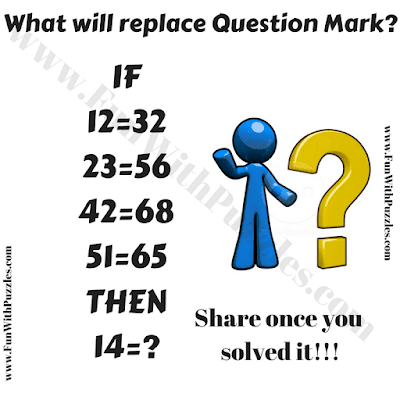Here is the Picture Number Puzzle for school going teens. In this Picture Number Puzzle, some number equations are given in the puzzle picture. Each of these number equation is logical correct with the same formula. Your challenge is to find this relationship among the given number in each equation and then find the missing number which will solve the last equation. Can you solve this picture number puzzle?Can you solve this Picture Number Puzzle?

The answer to this "Picture Number Puzzle for Teens", can be viewed by clicking on the button. Please do give your best try before looking at the answer.

Unknown said...